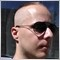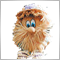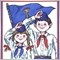# Requests & Ideas (MQL5 only!) - page 35212

The variable name is a constant. The name of the variable is unchanged.

I found a clear use case example:

```\$Reno = 360000;
\$cityname = "Reno";
echo "The size of \$cityname is \${\$cityname}";
echo "The size of \$cityname is \${\$cityname}";
//The output from this code is

//The size of Reno is 360000
//The size of Pasadena is 138000```

Will be my last attempt at explaining myself ;)[Deleted]

And why do we need such an indicator? What is the idea of ​​using it?

so we can know how we perform on the current day, either win or lose on trading.

that's big effect on trader emotion.Moderator
286047

forextime8 :

so we can know how we perform on the current day, either win or lose on trading.

that's big effect on trader emotion.

Where is the trading idea? What gives knowledge: today we are in profit or loss? How should this affect the trade of an adviser?37

I would like to modify this Fractal indicator that I attached so that I could modify the number of bars on side that has by default, this indicator that has the code open and can be modified, the number that I would like is 15/15, thanks

Files:Moderator
286047

eliot6 :

I would like to modify this Fractal indicator that I attached so that I could modify the  number of bars  on side that has by default, this indicator that has the code open and can be modified, the number that I would like is 15/15, thanks

Sorry, only MQL5 is discussed here. All questions on the old code you can solve in the service Freelance.359

huuh ...does i read correct -> this works in MT5 ?

`echo "The size of \$cityname is \${\$cityname}";`

if yes it would be amazing ....mmmmh more complex programming ...i could eat that language359

äähhmm i had a idea ... a nice c++ "try & catch" function would be a great feature :DModerator
286047

Christian Stern :

huuh ...does i read correct -> this works in MT5 ?

if yes it would be amazing ....mmmmh more complex programming ...i could eat that language

See the examples: PrintFormat

```void OnStart()
{
string server=AccountInfoString(ACCOUNT_SERVER);
//--- account number
//--- long value output
long leverage=AccountInfoInteger(ACCOUNT_LEVERAGE);
PrintFormat("%s %d: leverage = 1:%I64d",
//--- account currency
string currency=AccountInfoString(ACCOUNT_CURRENCY);
//--- double value output with 2 digits after the decimal point
double equity=AccountInfoDouble(ACCOUNT_EQUITY);
PrintFormat("%s %d: account equity = %.2f %s",
//--- double value output with mandatory output of the +/- sign
double profit=AccountInfoDouble(ACCOUNT_PROFIT);
PrintFormat("%s %d: current result for open positions = %+.2f %s",
//--- double value output with variable number of digits after the decimal point
double point_value=SymbolInfoDouble(_Symbol,SYMBOL_POINT);
string format_string=StringFormat("%%s: point value  = %%.%df",_Digits);
PrintFormat(format_string,_Symbol,point_value);
//--- int value output
PrintFormat("%s: current spread in points = %d ",
//--- double value output in the scientific (floating point) format with 17 meaningful digits after the decimal point
PrintFormat("DBL_MAX = %.17e",DBL_MAX);
//--- double value output in the scientific (floating point) format with 17 meaningful digits after the decimal point
PrintFormat("EMPTY_VALUE = %.17e",EMPTY_VALUE);
//--- output using PrintFormat() with default accuracy
PrintFormat("PrintFormat(EMPTY_VALUE) = %e",EMPTY_VALUE);
//--- simple output using Print()
Print("Print(EMPTY_VALUE) = ",EMPTY_VALUE);
/* execution result
MetaQuotes-Demo 1889998: leverage = 1:100
MetaQuotes-Demo 1889998: account equity = 22139.86 USD
MetaQuotes-Demo 1889998: current result for open positions = +174.00 USD
EURUSD: point value  = 0.00001
EURUSD: current spread in points = 12
DBL_MAX = 1.79769313486231570e+308
EMPTY_VALUE = 1.79769313486231570e+308
PrintFormat(EMPTY_VALUE) = 1.797693e+308
Print(EMPTY_VALUE) = 1.797693134862316e+308
*/
}```359

See the examples: PrintFormat

hey, no the point of using dynamic variables is, that i want call a list of classes and store them in a array. Now i use a construct of if conditions with hard coded class names. but i would be fancy to have a array of classnames and than call them such as:

```\$class = "foobar";

\${ \$class } myclass = new \${ \$class }();```

this is a very simple example without a loop and without a base class ( class interface )

i use this in perl to load modules for example. but i had this discussion in another thread and i guess it's not posible because the var names are static.Moderator
286047

Christian Stern :

hey, no the point of using dynamic variables is, that i want call a list of classes and store them in a array. Now i use a construct of if conditions with hard coded class names. but i would be fancy to have a array of classnames and than call them such as:

this is a very simple example without a loop and without a base class ( class interface )

i use this in perl to load modules for example. but i had this discussion in another thread and i guess it's not posible because the var names are static.

That's right, in MQL5  the var names are static.